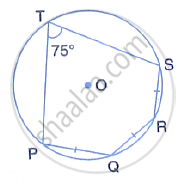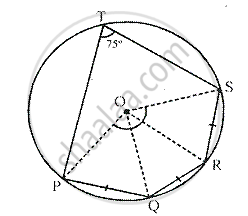Share

# The Given Figure Shows a Circle with Centre O. Also, Pq = Qr = Rs and ∠Pts = 75°. Calculate: (I) ∠Pos, (Ii) ∠Qor, (Iii) ∠Pqr. - Mathematics

Course

#### Question

The given figure shows a circle with centre O. Also, PQ = QR = RS and ∠PTS = 75°. Calculate:
(i) ∠POS,  (ii) ∠QOR,  (iii) ∠PQR.#### SolutionJoin OP, OQ and OS.

∵  PQ = QR = RS,
∠POQ = ∠QOR = ∠ROS

[Equal chords subtends equal angles at the centre]

Arc PQRS subtends ∠POS at the center and ∠PTS at the remaining Parts of the circle.
∴ ∠POS = 2∠PTS = 2 × 75° = 150°
⇒ ∠POQ + ∠QOR + ∠ROS = 150°
⇒ ∠POQ = ∠QOR = ∠ROS = (150° )/3 = 50°
In Δ OPQ, OP = OQ                [radii of the same circle]
∴ ∠OPQ = ∠OQP

But ∠OQP + ∠POQ = 180°

∴ ∠OPQ + ∠QP = 50° = 180°
⇒ ∠OPQ + ∠OQP = 180°  - 50°
⇒ ∠OPQ + ∠OPQ = 130°
⇒  2∠OPQ = 130°
⇒ ∠OPQ = ∠OQP = (130°)/2  = 65°
Similarly, we can prove that
In ΔOQR, ∠OQR = ∠ORQ = 65°
And in ΔORS, ∠ORS = OSR = 65°
(i) Now ∠POS = 150°
(ii) QOR = 50° and
(iii) ∠PQR = ∠PQO + ∠OQR = 65° + 65° = 130°

Is there an error in this question or solution?